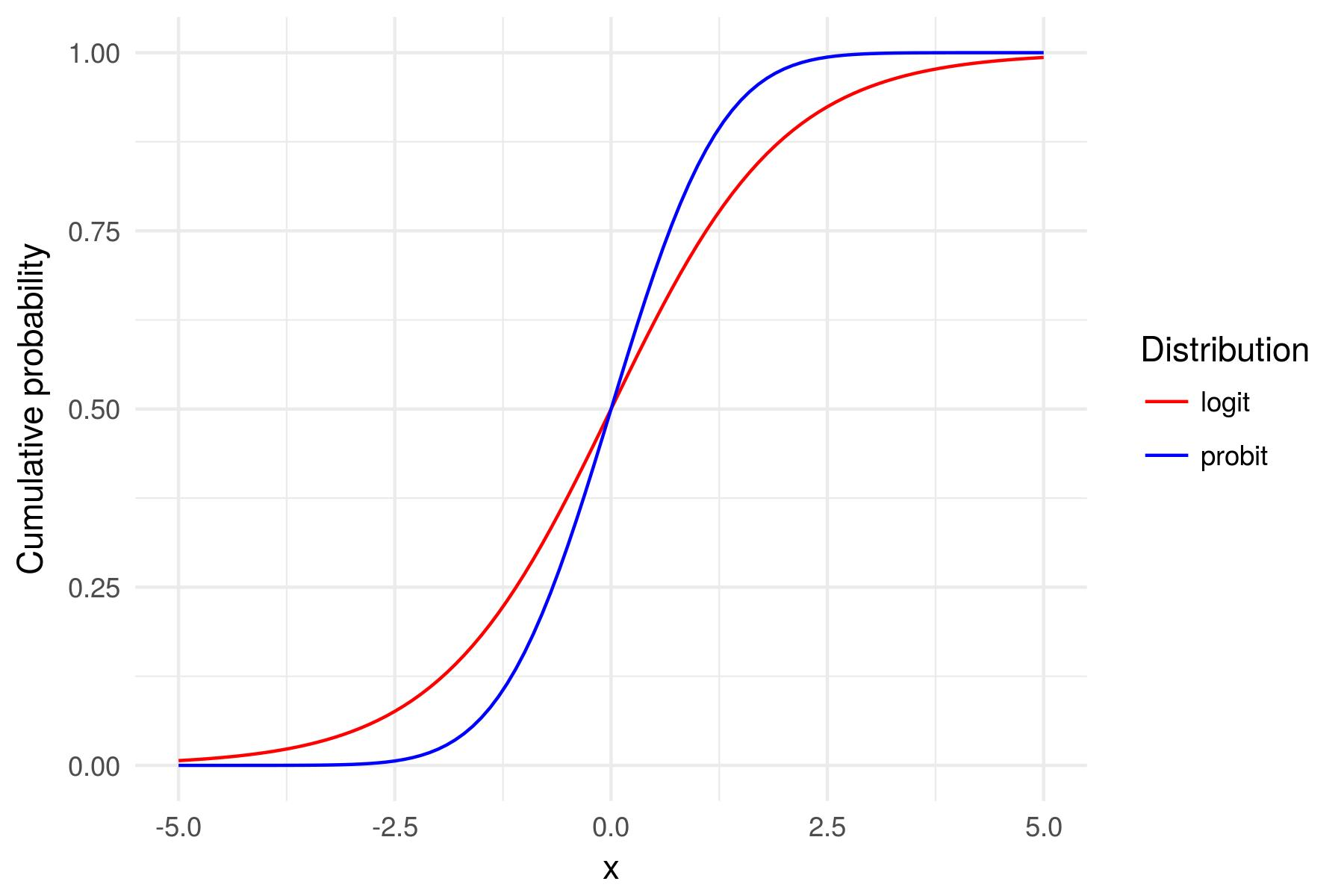# Probit versus logit

In the video, you learned about probit and logistic regression. In figure are a logit and probit curve. The x-axis is the input value (i.e., model input x) and the y-axis is the probability corresponding to the input.